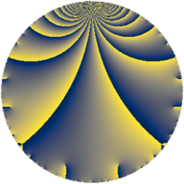# Properties

 Label 4046.2.a.fLevel $4046$ Weight $2$ Character orbit 4046.a Self dual yes Analytic conductor $32.307$ Analytic rank $1$ Dimension $1$ CM no Inner twists $1$

# Related objects

## Newspace parameters

 Level: $$N$$ $$=$$ $$4046 = 2 \cdot 7 \cdot 17^{2}$$ Weight: $$k$$ $$=$$ $$2$$ Character orbit: $$[\chi]$$ $$=$$ 4046.a (trivial)

## Newform invariants

 Self dual: yes Analytic conductor: $$32.3074726578$$ Analytic rank: $$1$$ Dimension: $$1$$ Coefficient field: $$\mathbb{Q}$$ Coefficient ring: $$\mathbb{Z}$$ Coefficient ring index: $$1$$ Twist minimal: no (minimal twist has level 14) Fricke sign: $$1$$ Sato-Tate group: $\mathrm{SU}(2)$

## $q$-expansion

 $$f(q)$$ $$=$$ $$q - q^{2} + 2q^{3} + q^{4} - 2q^{6} - q^{7} - q^{8} + q^{9} + O(q^{10})$$ $$q - q^{2} + 2q^{3} + q^{4} - 2q^{6} - q^{7} - q^{8} + q^{9} + 2q^{12} - 4q^{13} + q^{14} + q^{16} - q^{18} + 2q^{19} - 2q^{21} - 2q^{24} - 5q^{25} + 4q^{26} - 4q^{27} - q^{28} + 6q^{29} + 4q^{31} - q^{32} + q^{36} - 2q^{37} - 2q^{38} - 8q^{39} - 6q^{41} + 2q^{42} + 8q^{43} - 12q^{47} + 2q^{48} + q^{49} + 5q^{50} - 4q^{52} + 6q^{53} + 4q^{54} + q^{56} + 4q^{57} - 6q^{58} - 6q^{59} - 8q^{61} - 4q^{62} - q^{63} + q^{64} - 4q^{67} - q^{72} - 2q^{73} + 2q^{74} - 10q^{75} + 2q^{76} + 8q^{78} - 8q^{79} - 11q^{81} + 6q^{82} - 6q^{83} - 2q^{84} - 8q^{86} + 12q^{87} - 6q^{89} + 4q^{91} + 8q^{93} + 12q^{94} - 2q^{96} + 10q^{97} - q^{98} + O(q^{100})$$

## Embeddings

For each embedding $$\iota_m$$ of the coefficient field, the values $$\iota_m(a_n)$$ are shown below.

For more information on an embedded modular form you can click on its label.

Label $$\iota_m(\nu)$$ $$a_{2}$$ $$a_{3}$$ $$a_{4}$$ $$a_{5}$$ $$a_{6}$$ $$a_{7}$$ $$a_{8}$$ $$a_{9}$$ $$a_{10}$$
1.1
 0
−1.00000 2.00000 1.00000 0 −2.00000 −1.00000 −1.00000 1.00000 0
 $$n$$: e.g. 2-40 or 990-1000 Significant digits: Format: Complex embeddings Normalized embeddings Satake parameters Satake angles

## Atkin-Lehner signs

$$p$$ Sign
$$2$$ $$1$$
$$7$$ $$1$$
$$17$$ $$1$$

## Inner twists

This newform does not admit any (nontrivial) inner twists.

## Twists

By twisting character orbit
Char Parity Ord Mult Type Twist Min Dim
1.a even 1 1 trivial 4046.2.a.f 1
17.b even 2 1 14.2.a.a 1
51.c odd 2 1 126.2.a.b 1
68.d odd 2 1 112.2.a.c 1
85.c even 2 1 350.2.a.f 1
85.g odd 4 2 350.2.c.d 2
119.d odd 2 1 98.2.a.a 1
119.h odd 6 2 98.2.c.a 2
119.j even 6 2 98.2.c.b 2
136.e odd 2 1 448.2.a.a 1
136.h even 2 1 448.2.a.g 1
153.h even 6 2 1134.2.f.l 2
153.i odd 6 2 1134.2.f.f 2
187.b odd 2 1 1694.2.a.e 1
204.h even 2 1 1008.2.a.h 1
221.b even 2 1 2366.2.a.j 1
221.g odd 4 2 2366.2.d.b 2
255.h odd 2 1 3150.2.a.i 1
255.o even 4 2 3150.2.g.j 2
272.k odd 4 2 1792.2.b.g 2
272.r even 4 2 1792.2.b.c 2
323.c odd 2 1 5054.2.a.c 1
340.d odd 2 1 2800.2.a.g 1
340.r even 4 2 2800.2.g.h 2
357.c even 2 1 882.2.a.i 1
357.q odd 6 2 882.2.g.c 2
357.s even 6 2 882.2.g.d 2
391.c odd 2 1 7406.2.a.a 1
408.b odd 2 1 4032.2.a.w 1
408.h even 2 1 4032.2.a.r 1
476.e even 2 1 784.2.a.b 1
476.o odd 6 2 784.2.i.c 2
476.q even 6 2 784.2.i.i 2
595.b odd 2 1 2450.2.a.t 1
595.p even 4 2 2450.2.c.c 2
952.e odd 2 1 3136.2.a.e 1
952.k even 2 1 3136.2.a.z 1
1428.b odd 2 1 7056.2.a.bd 1

By twisted newform orbit
Twist Min Dim Char Parity Ord Mult Type
14.2.a.a 1 17.b even 2 1
98.2.a.a 1 119.d odd 2 1
98.2.c.a 2 119.h odd 6 2
98.2.c.b 2 119.j even 6 2
112.2.a.c 1 68.d odd 2 1
126.2.a.b 1 51.c odd 2 1
350.2.a.f 1 85.c even 2 1
350.2.c.d 2 85.g odd 4 2
448.2.a.a 1 136.e odd 2 1
448.2.a.g 1 136.h even 2 1
784.2.a.b 1 476.e even 2 1
784.2.i.c 2 476.o odd 6 2
784.2.i.i 2 476.q even 6 2
882.2.a.i 1 357.c even 2 1
882.2.g.c 2 357.q odd 6 2
882.2.g.d 2 357.s even 6 2
1008.2.a.h 1 204.h even 2 1
1134.2.f.f 2 153.i odd 6 2
1134.2.f.l 2 153.h even 6 2
1694.2.a.e 1 187.b odd 2 1
1792.2.b.c 2 272.r even 4 2
1792.2.b.g 2 272.k odd 4 2
2366.2.a.j 1 221.b even 2 1
2366.2.d.b 2 221.g odd 4 2
2450.2.a.t 1 595.b odd 2 1
2450.2.c.c 2 595.p even 4 2
2800.2.a.g 1 340.d odd 2 1
2800.2.g.h 2 340.r even 4 2
3136.2.a.e 1 952.e odd 2 1
3136.2.a.z 1 952.k even 2 1
3150.2.a.i 1 255.h odd 2 1
3150.2.g.j 2 255.o even 4 2
4032.2.a.r 1 408.h even 2 1
4032.2.a.w 1 408.b odd 2 1
4046.2.a.f 1 1.a even 1 1 trivial
5054.2.a.c 1 323.c odd 2 1
7056.2.a.bd 1 1428.b odd 2 1
7406.2.a.a 1 391.c odd 2 1

## Hecke kernels

This newform subspace can be constructed as the intersection of the kernels of the following linear operators acting on $$S_{2}^{\mathrm{new}}(\Gamma_0(4046))$$:

 $$T_{3} - 2$$ $$T_{5}$$ $$T_{23}$$

## Hecke characteristic polynomials

$p$ $F_p(T)$
$2$ $$1 + T$$
$3$ $$-2 + T$$
$5$ $$T$$
$7$ $$1 + T$$
$11$ $$T$$
$13$ $$4 + T$$
$17$ $$T$$
$19$ $$-2 + T$$
$23$ $$T$$
$29$ $$-6 + T$$
$31$ $$-4 + T$$
$37$ $$2 + T$$
$41$ $$6 + T$$
$43$ $$-8 + T$$
$47$ $$12 + T$$
$53$ $$-6 + T$$
$59$ $$6 + T$$
$61$ $$8 + T$$
$67$ $$4 + T$$
$71$ $$T$$
$73$ $$2 + T$$
$79$ $$8 + T$$
$83$ $$6 + T$$
$89$ $$6 + T$$
$97$ $$-10 + T$$